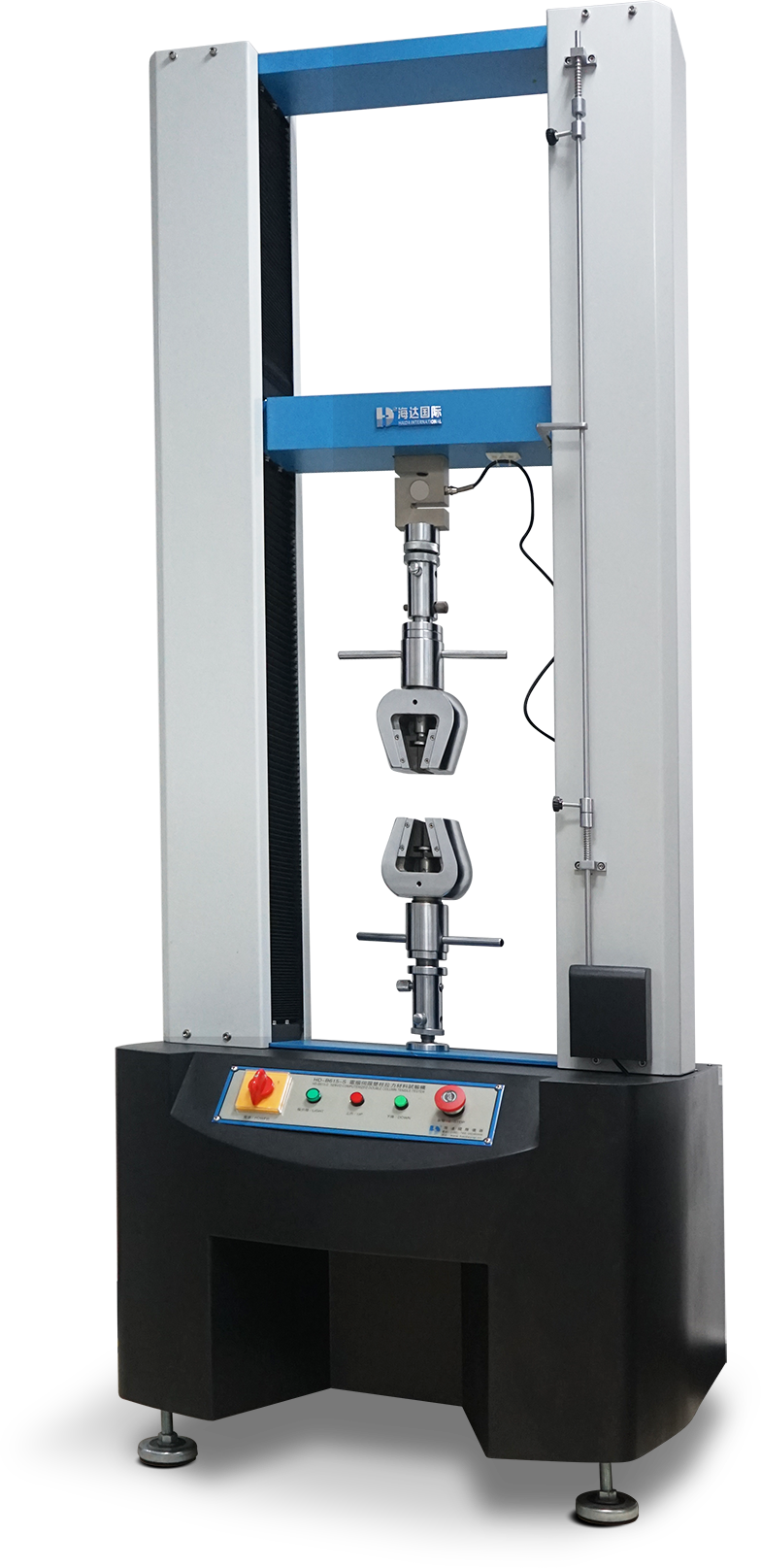# Tensile strength test of universal testing machine

Views:0     Author:Site Editor     Publish Time: 2021-08-24      Origin:SiteThe universal testing machine has a wide range of test items. The main test items are: tensile stress, tensile strength, constant elongation stress, constant stress elongation, breaking strength, elongation after fracture, yield strength, elongation at yield point , Yield point tensile stress, tear strength, peel strength, puncture strength, bending strength, elastic modulus, etc. How are the test items defined? Here is a brief description of the characteristics of each major project test:

Tensile strength test:

A brief introduction to the main test items of the universal testing machine:

1. Tensile stress definition: the stress generated by the sample during stretching. Its value is the ratio of the applied force to the original cross-sectional area of the sample, in MPa. In rubber tensile testing, a dumbbell-shaped test is often used. It can also be a ring test piece;

2. Definition of tensile strength: also known as tensile strength, which refers to the maximum tensile stress that the sample is subjected to until it breaks in a tensile test, which is the tensile strength, and the unit of strength is MPa;

3. Tensile stress at a given elongation, definition: refers to the tensile test of the rubber material, the tensile stress when the working part of the sample is stretched to a given elongation, and the common tensile stress at a given elongation;

4. Elongation at a given stress, definition: the elongation when the sample is stretched to a given stress;

5. Breaking strength definition: refers to the stress at which a material breaks, a linear load is applied in the diameter direction of the sample, and the strength when the sample reaches failure, the unit is Mpa;

6. Percentage elongation after fracture Definition: In the tensile test, the relative elongation value of the gauge length after the specimen is broken is equal to the absolute elongation of the gauge length divided by the gauge length of the specimen before the tension;

7. The definition of yield strength: also known as the yield limit, it is the yield limit when a metal material yields, that is, the stress that resists a small amount of plastic deformation. For metal materials with no obvious yield, it is stipulated that the stress value that produces 0.2% residual deformation is the yield limit, which is called the conditional yield limit or yield strength;

8. Elongation at yield, definition: refers to the elongation corresponding to the yield point of the sample;

9. Tensile stress at yield point, definition: refers to the transition point between the "Hooker zone" and the yield zone on the tensile curve is called the yield point, and the stress and strain corresponding to the yield point are respectively called the yield point stress And yield point strain;

10. Tearing strength, definition: refers to the stress when the test film sheet or rubber plastic material is torn;

11. Peel strength, definition: refers to the maximum load required per unit width when the two adherends of the adhesive are separated under the specified peeling conditions;

12. Bending strength, definition: the maximum stress that a material can withstand when it ruptures under a bending load or reaches a specified deflection. It is mainly used to test the performance of the material when it is subjected to a bending load;

13. Shear strength, definition: refers to the strength limit when the external force is perpendicular to the material axis and acts on the material in shear. It is mainly used to test the ability of the material to withstand the shear force;

14. Elastic modulus, definition: also known as Young's modulus, refers to the ratio of the normal stress to the corresponding normal strain of the material in the elastic deformation stage;

Application of main test items of universal testing machine:

Universal testing machines are generally used for photovoltaic materials such as rubber, plastics, films, adhesives, steel bars, glass, touch screens, textiles, waterproof materials, wires and cables, mesh ropes, metal wires, metal rods, metal plates, and clothing industries.